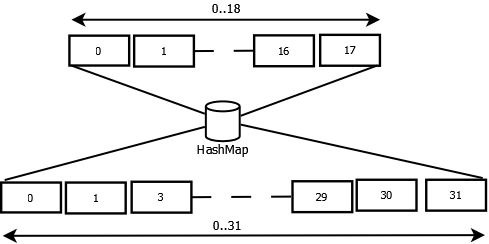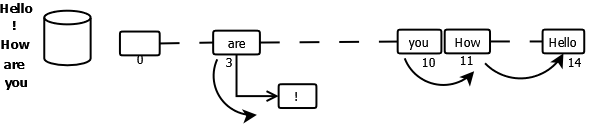## Internal working of HashMap

A hash map is a data structure that uses a hash function to map keys to their associated values. This article describes the internal workings of a HashMap. The main source of the article is java source code.

For Q&A, visit HashMap Q&A.

## Data Structure

In HashMap, the data structure is based on array and linked list. An entry finds its location in the array based on its hash value. If an array element is already occupied, the new entry replaces the old entry and the old entry is linked to the new one. Below is an example of the entries in the data structure.

## Put Entry

When a new entry is assigned to an index, the existing entry to the index, if any, becomes the next entry to the new entry.

## Entry

An Entry consists of key, value, hash and the next entry sharing the index.

## Capacity

The default initial capacity MUST be a power-of-two. Even if the caller provides a capacity c which is 2x-1 > c < 2x, the capacity is converted to 2x to create the internal array.Capacity Adjustment to match power-of-two

If the capacity of array is in power-of-two, the hash code can be easily converted to the index based on a simple AND operation:

`index =  hashCode & (length-1)`

Here length = 2x.

In Hashtable, the capacity doesn’t need to be in power of 2 and the internal array is created for whatever capacity is provided but the index calculation is based on modulus operand:

`index = (hashCode & 0x7FFFFFFF) % length.`

Since the capacity is in power of 2, a simple AND operation is able to return us the index.

Lets see how it works using an example.

Suppose the hash=311 and length=16, applying the modulus operation we get:
hash = 16 * 19 + 7 (length*quotient + index), thus index = 7.

Let us convert the hash to binary.
311 = 1 0011 0111 in binary
= 1 0011 0111 = 1 0011 0000 + 0111
= 24*1 + 25*1 + 26*0 + 27*0 + 28*1 + 0111
= 24(20*1 + 21*1 + 22*0 + 23*0 + 24*1) + 0111
= 24(10011) + 0111

This is in the same form as the length*quotient + index, here index = 0111, thus if length=2x, index is nothing but the lower x bits of hash code.

We can obtain these lower bits by doing & operation.

index = 0111 = 1 0111 0111 & 1111

Thus index = hash & (2length – 1).

```public void testFindModulusByAND() {
int n = 311;
int d = 1 << 4;
int m = 7;
assertEquals(m, n & (d -1));
}```

## Re-hashing

Since HashMap uses power-of-two length hash table, the lower bits form the index. If the hash function is poor, it may lead to different hash codes but with same lower bits.

If a hash code results into the same index, we encounter collisions. In order to avoid this, HashMap applies a supplemental hash function to a given hashCode. For example, hash codes 375 and 311, has the same lower bits so they return the same index.

375 = 1 0111 0111
311 = 1 0011 0111

The index would be the lower four bits 0111=7.
HashMap applies the below hash function to refine the original hash code.

``` h ^= (h >>> 20) ^ (h >>> 12);
h ^= (h >>> 7) ^ (h >>> 4);```

When we apply it on 311, we get 294=1 0010 0110, index is 6.
When we apply it on 375, we get 354=1 0110 0010, index is 2.

## Collision

If two different key objects return the same hashcode, it will result in collision as the bucket location would be same. The new entry will replace the previous entry and the next reference of the new entry will point to the previous entry. For hash tables, this means that the second table insertion will be slower as the links have to be adjusted.

## Get Entry

The key is converted to its hash value and then HashMap’s internal hash function is applied on it to get the actual hash code. This hash code is then used to identify the bucket.

The Object sitting in the bucket is of type Entry so it knows the key, value, hash and its next entry, if any. There may be more than one object stored in same bucket, in such case, the entry will be a linked list where next entry will be its next node.

We must traverse through the list to identify the Object for the associated key. If the entry’s hash matches with key’s hash value and its key matches with the key in context, it means we have found the correct node.

1. e.hash == hash
2. e.key == key || key.equals(k)

It is important that the key used in HashMap should be immutable, final object with proper equals() and hashcode() implementation.

## NULL Key

HashMap accepts Null key, index 0 is reserved for the Null key entry.

## Re-sizing

### Threshold

Threshold defines the next size value at which to resize the hash table. If the size of the map exceeds a given threshold defined by load-factor e.g. if load factor is .75, it will act to re-size the map once it filled 75%. Java HashMap does that by creating another new bucket array of size twice of previous size of HashMap. The new entry can occupy any index based on the hash value.

In the below diagram, the emphasis is on the relation between size, threshold and expansion of capacity. In order to simplify the diagram, it is assumed the new entries occupy array elements in serial order.

All the entries are transferred from current table to new Table.

This process is called rehashing because it also applies hash function to find new bucket location.

### Transfer of HashMap elements

Whenever we have to re-size the hash table, the elements from the old array will have to be transferred to the new expanded array. Since the capacity is doubled, the elements based on the hash key may either stay at same index, or move with a power of two offset.

## Iterator

Since the hash value determines the bucket location, the iterator doesn’t promises any order. Iterator returns the first not null element in the array starting with index=0.
Below example puts keys in order “Hello”,”!”, “How”,”are”,”you” to a HashMap object but iterator returns in completely different order. It returns elements from the first available entry, its next entry in the linked list, if any, else the next available entry in the table till it reaches the end of the table.Order of elements returned by the iterator

### Iterator Example

```    public void testMapItr() {
Map m = new HashMap();
m.put("Hello", null);
m.put("!", null);
m.put("How", null);
m.put("are", null);
m.put("you", null);
Iterator itr = m.keySet().iterator();
while (itr.hasNext()) {
String key = itr.next();
int hash = hash(key.hashCode());
System.out.println("table[" + (hash & 15) + "]=" + key);
}
}
static int hash(int h) {
h ^= (h >>> 20) ^ (h >>> 12);
return h ^ (h >>> 7) ^ (h >>> 4);
}```

Result:

```table=are
table=!
table=you
table=How
table=Hello```

## More Java Maps

There are more articles on Java Maps that you may want to read here:

Share.